##### Statistics: 1001 Practice Problems For Dummies (+ Free Online Practice)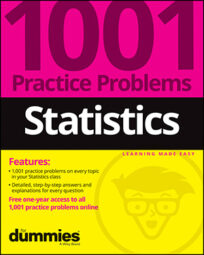Descriptive statistics are statistics that describe data. Two of the staple ingredients of descriptive statistics are the mean and median. Your first job in analyzing data is to identify, understand, and calculate these descriptive statistics. Solve the following problems about means and medians.

## Sample questions

1. To the nearest tenth, what is the mean of the following data set? 14, 14, 15, 16, 28, 28, 32, 35, 37, 38

Use the formula for calculating the mean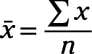where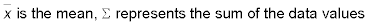and n is the number of values in the data set.

In this case, x = 14 + 14 + 15 + 16 + 28 + 28 + 32 + 35 + 37 + 38 = 257, and n = 10. So the mean is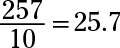2. To the nearest tenth, what is the mean of the following data set? 0.8, 1.8, 2.3, 4.5, 4.8, 16.1, 22.3

Use the formula for calculating the mean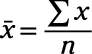whereand n is the number of values in the data set.

In this case, x = 0.8 + 1.8 + 2.3 + 4.5 + 4.8 + 16.1 + 22.3 = 52.6, and n = 7. So the mean is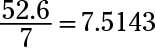The question asks for the nearest tenth, so you round to 7.5.

3. To the nearest tenth, what is the median of the following data set? 6, 12, 22, 18, 16, 4, 20, 5, 15

To find the median, put the numbers in order from smallest to largest:

4, 5, 6, 12, 15, 16, 18, 20, 22

Because this data set has an odd number of values (nine), the median is simply the middle number in the data set: 15.

4. To the nearest tenth, what is the median of the following data set? 14, 2, 21, 7, 30, 10, 1, 15, 6, 8

To find the median, put the numbers in order from smallest to largest:

1, 2, 6, 7, 8, 10, 14, 15, 21, 30

Because this data set has an even number of values (ten), the median is the average of the two middle numbers: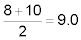5. Compare the mean and median of a data set that has a distribution that is skewed right.

Answer: The mean will have a higher value than the median.

A data set distribution that is skewed right is asymmetrical and has a large number of values at the lower end and few numbers at the high end. In this case, the median, which is the middle number when you sort the data from smallest to largest, lies in the lower range of values (where most of the numbers are).

However, because the mean finds the average of all the values, both high and low, the few outlying data points on the high end cause the mean to increase, making it higher than the median.

6. Compare the mean and the median of a data set that has a symmetrical distribution.

Answer: The mean and median will be fairly close together.

When a data set has a symmetrical distribution, the mean and the median are close together because the middle value in the data set, when ordered smallest to largest, resembles the balancing point in the data, which occurs at the average.

If you need more practice on this and other topics from your statistics course, visit 1,001 Statistics Practice Problems For Dummies to purchase online access to 1,001 statistics practice problems! We can help you track your performance, see where you need to study, and create customized problem sets to master your stats skills.